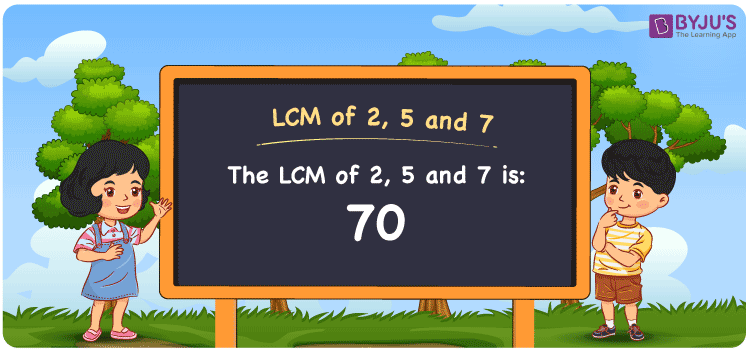# LCM of 2, 5 and 7

LCM of 2, 5 and 7 is 70. We can obtain the least common multiple of 2, 5 and 7 with ease from the common multiples of each number. (2, 4, 6, 8, 10, 12, 14, 16, ….), (5, 10, 15, 20, 25, 30, 35,…..) and (7, 14, 21, 28, 35, 42, ….) are the multiples of 2, 5 and 7. Prime factorisation, division and by listing the multiples are the methods to find the LCM of two numbers.

Also read: Least common multiple

## What is LCM of 2, 5 and 7?

The answer to this question is 70. The LCM of 2, 5 and 7 using various methods is shown in this article for your reference. The LCM of two non-zero integers, 2, 5 and 7, is the smallest positive integer 70 which is divisible by both 2, 5 and 7 with no remainder.## How to Find LCM of 2, 5 and 7?

LCM of 2, 5 and 7 can be found using three methods:

• Prime Factorisation
• Division method
• Listing the multiples

### LCM of 2, 5 and 7 Using Prime Factorisation Method

The prime factorisation of 2, 5 and 7, respectively, is given by:

2 = 2¹

5 = 5¹

7 = 7¹

LCM (2, 5, 7) = 70

### LCM of 2, 5 and 7 Using Division Method

We’ll divide the numbers (2, 5, 7) by their prime factors to get the LCM of 2, 5 and 7 using the division method (preferably common). The LCM of 2, 5 and 7 is calculated by multiplying these divisors.

 2 2 5 7 5 1 5 7 7 1 1 7 x 1 1 1

No further division can be done.

Hence, LCM (2, 5, 7) = 70

### LCM of 2, 5 and 7 Using Listing the Multiples

To calculate the LCM of 2, 5 and 7 by listing out the common multiples, list the multiples as shown below

• Multiples of 2 = 2, 4, 6, 8, 10, 12, 14, 16, 18, 20, . . . ., 66, 68, 70, . . . .
• Multiples of 5 = 5, 10, 15, 20, 25, 30, 35, 40, 45, 50, . . . ., 55, 60, 65, 70, . . . .
• Multiples of 7 = 7, 14, 21, 28, 35, 42, 49, 56, 63, 70, . . . ., 49, 56, 63, 70, . . . .

The smallest common multiple of 2, 5 and 7 is 70.

Therefore, LCM (2, 5, 7) = 70

## Video Lesson on Applications of LCM## LCM of 2, 5 and 7 Solved Examples

Question: Find the LCM of 2, 5 and 7 in the numbers 40, 50, 25, 70.

Solution:

LCM is the smallest common multiple divisible exactly by the given numbers

The number 70 satisfies this condition

Hence, the LCM is 70.

## Frequently Asked Questions on LCM of 2, 5 and 7

### How to find the LCM of 2, 5 and 7?

We know that the LCM of 2, 5 and 7 is 70. To find the LCM, we have to know the multiples and the smallest multiple which is divisible by 2, 5 and 7 has to be calculated.

### Write the methods to get the LCM of 2, 5 and 7.

The methods to get the LCM of 2, 5 and 7 are the Division Method, Prime Factorization Method and Listing multiples.

### If the LCM of 2, 5 and 7 is 70, what is the GCF?

LCM x GCF = 2 x 5 x 7

Given

LCM of 2, 5 and 7 = 70

70 x GCF = 70

GCF = 70/70 = 1

### What is the LCM of 2, 5 and 7?

The LCM of 2, 5 and 7 is 70.

### Show the relation between GCF and LCM of 2, 5 and 7.

The relation between GCF and LCM of 2, 5 and 7 is

GCF x LCM = 2 x 5 x 7

GCF x LCM = 70